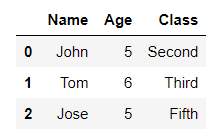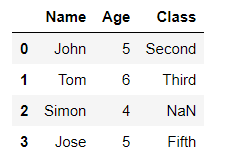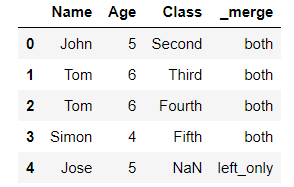# Merge two Pandas DataFrames with complex conditions

• Last Updated : 07 Apr, 2021

In this article, we let’s discuss how to merge two Pandas Dataframe with some complex conditions. Dataframes in Pandas can be merged using pandas.merge() method.

Syntax:

Attention geek! Strengthen your foundations with the Python Programming Foundation Course and learn the basics.

To begin with, your interview preparations Enhance your Data Structures concepts with the Python DS Course. And to begin with your Machine Learning Journey, join the Machine Learning - Basic Level Course

pandas.merge(parameters)

Returns : A DataFrame of the two merged objects.

While working on datasets there may be a need to merge two data frames with some complex conditions, below are some examples of merging two data frames with some complex conditions.

Example 1 :

Merging two data frames with merge() function with the parameters as the two data frames.

## Python3

 `# importing pandas as pd``import` `pandas as pd`` ` `# creating dataframes``df1 ``=` `pd.DataFrame({``'ID'``: [``1``, ``2``, ``3``, ``4``], ``                    ``'Name'``: [``'John'``, ``'Tom'``, ``'Simon'``, ``'Jose'``]})`` ` `df2 ``=` `pd.DataFrame({``'ID'``: [``1``, ``2``, ``3``, ``5``], ``                    ``'Class'``: [``'Second'``, ``'Third'``, ``'Fifth'``, ``'Fourth'``]})`` ` `# merging df1 and df2 with merge function``df ``=` `pd.merge(df1, df2)``print``(df)`

Output :Example 2 :

Merging two data frames with merge() function on some specified column name of the data frames.

## Python3

 `# importing pandas as pd``import` `pandas as pd`` ` `# creating dataframes``df1 ``=` `pd.DataFrame({``'Name'``: [``'John'``, ``'Tom'``, ``'Simon'``, ``'Jose'``],``                    ``'Age'``: [``5``, ``6``, ``4``, ``5``]})`` ` `df2 ``=` `pd.DataFrame({``'Name'``: [``'John'``, ``'Tom'``, ``'Jose'``],``                    ``'Class'``: [``'Second'``, ``'Third'``, ``'Fifth'``]})`` ` `# merging df1 and df2 with merge function ``# with the common column as Name``df ``=` `pd.merge(df1, df2, on``=``'Name'``)``print``(df)`

Output :Example 3 :

Merging two data frames with all the values in the first data frame and NaN for the not matched values from the second data frame. The same can be done to merge with all values of the second data frame what we have to do is just give the position of the data frame when merging as left or right.

## Python3

 `# importing pandas as pd``import` `pandas as pd`` ` `# creating dataframes``df1 ``=` `pd.DataFrame({``'Name'``: [``'John'``, ``'Tom'``, ``'Simon'``, ``'Jose'``], ``                    ``'Age'``: [``5``, ``6``, ``4``, ``5``]})`` ` `df2 ``=` `pd.DataFrame({``'Name'``: [``'John'``, ``'Tom'``, ``'Jose'``],``                    ``'Class'``: [``'Second'``, ``'Third'``, ``'Fifth'``]})`` ` `# merging df1 and df2 with merge function ``# with the common column as Name``df ``=` `pd.merge(df1, df2, on``=``'Name'``, how``=``"left"``)``print``(df)`

Output :Example 4 :

Merging two data frames with all the values of both the data frames using merge function with an outer join. The same can be done do join two data frames with inner join as well.

## Python3

 `# importing pandas as pd``import` `pandas as pd`` ` `# creating dataframes``df1 ``=` `pd.DataFrame({``'Name'``:[``'John'``, ``'Tom'``, ``'Simon'``, ``'Jose'``],``                    ``'Age'``:[``5``, ``6``, ``4``, ``5``]})`` ` `df2 ``=` `pd.DataFrame({``'Name'``:[``'John'``, ``'Tom'``, ``'Philip'``],``                    ``'Class'``:[``'Second'``, ``'Third'``, ``'Fifth'``]})`` ` `# merging df1 and df2 with merge function ``# with the records of both the dataframes``df ``=` `pd.merge(df1, df2, how ``=` `"outer"``)``print``(df)`

Output :Example 5 :

Merging data frames with the indicator value to see which data frame has that particular record.

## Python3

 `# importing pandas as pd``import` `pandas as pd`` ` `# creating dataframes``df1 ``=` `pd.DataFrame({``'Name'``:[``'John'``, ``'Tom'``, ``'Simon'``, ``'Jose'``],``                    ``'Age'``:[``5``, ``6``, ``4``, ``5``]})`` ` `df2 ``=` `pd.DataFrame({``'Name'``:[``'John'``, ``'Tom'``, ``'Simon'``, ``'Tom'``],``                    ``'Class'``:[``'Second'``, ``'Third'``, ``'Fifth'``, ``'Fourth'``]})`` ` `# merging df1 and df2 with merge function ``# with the records of both the dataframes``df ``=` `pd.merge(df1, df2, how ``=` `'left'``, indicator ``=` `True``)``print``(df)`

Output :Example 6 :

Merging data frames with the one-to-many relation in the two data frames. The same can be done to merge with many-to-many, one-to-one, and one-to-many type of relationship.

## Python3

 `# importing pandas as pd``import` `pandas as pd`` ` `# creating dataframes``df1 ``=` `pd.DataFrame({``'Name'``:[``'John'``, ``'Tom'``, ``'Simon'``, ``'Jose'``],``                    ``'Age'``:[``5``, ``6``, ``4``, ``5``]})`` ` `df2 ``=` `pd.DataFrame({``'Name'``:[``'John'``, ``'Tom'``, ``'Simon'``, ``'Tom'``],``                    ``'Class'``:[``'Second'``, ``'Third'``, ``'Fifth'``, ``'Fourth'``]})`` ` `# merging df1 and df2 with merge function with ``# the one to many relations from both the dataframes``df ``=` `pd.merge(df1, df2, validate ``=` `'one_to_many'``)``print``(df)`

Output :My Personal Notes arrow_drop_up